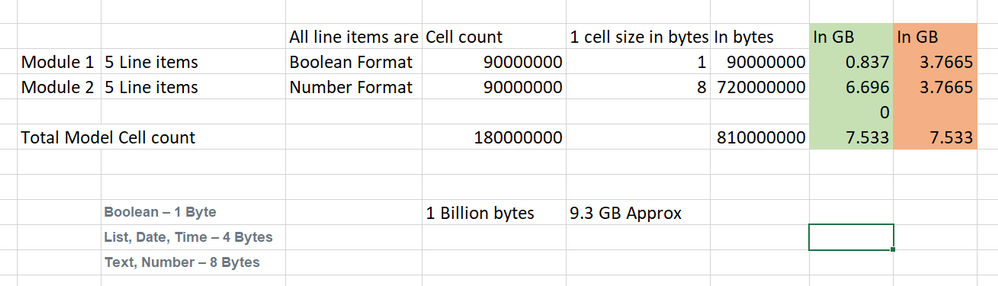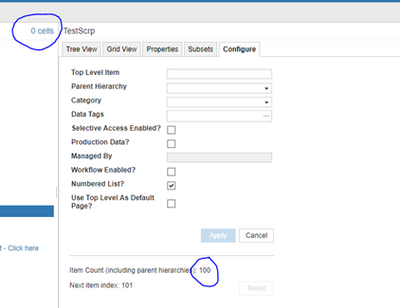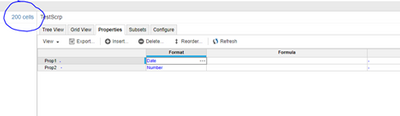# Calculation of modules & Size....Hi Everyone,

Can anyone help me to understand, how we are calculating the module size in GB format? If I want to see the actual size of my modules in GB then, do i need to enable any option in Anaplan.

Regards,,,

___________________________________________________________________________________________

Tagged:

•Hi @Pranit6,

Hope it helps,
Regards,

Gaëtan

•This is a rough, high-level way to estimate - here's how I go about it: I get the total model size by going to the gear and double Cartoon HD clicking on the cell count that shows up next to the "Model Settings" header (you can also get the model size by going to "Manage Models" and it will be displayed by the model name) I get the total cell count either from the same location (rounded) or Vidmate by adding up the cell counts from each module Divide the model size by the number of model cells to get a size per cell To calculate the size of a given module, multiply the cell count from that module times the model size per cell from above Again - this is rough and does leave out the size of lists (which may or may not be material, depending on how your model is set up) and possibly other factors as well. But it's a good way to get an estimate. 9Apps

•• 48 bytes per non-empty cell + 2 bytes per character

Which is why in David's planual (nice work, by the way) he mentions to avoid text when possible.

•This is a rough, high-level way to estimate - here's how I go about it: I get the total model size by going to the gear and double clicking on the cell count that shows up next to the "Model Settings" header (you can also get the model size by going to "Manage Models" and it will be displayed by the model name) I get the total cell count either from the same location (rounded) or by adding up the cell counts from each module Divide the model size by the number of model cells to get a size per cell To calculate the size of a given module, multiply the cell count from that module times the model size per cell from above Again - this is rough and does leave out the size of lists (which may or may not be material, depending on how your model is set up) and possibly other factors as well. But it's a good way to get an estimate.
•Try this to get almost perfect size calculation for each module.

Module 1 has cell count of 90M and boolean format, the Actual size is 0.83 GB, as per yours 3.7 GB

Module 2 has cell count of 90M and Number format, the Actual size is 6.6 GB, as per yours 3.7 GBInformation provided above by David is the best way to calculate the accurate size.

Regards,

Vignesh M

•Thanks a lot for the detailed information, it is really helpful. However I found some anomalies between what you say and what I observed.

I created a list with exactly 100 List items in it - Cell Count didn't go up,it remained zero as you rightly mentionedI then added two properties to it, date and number formatted - Cell count jumped to 200. This is against what you said in your comment section."List Properties add to your space and not to cell count". Below screenshot for your refDid you intend to say list items? Let me know if I am missing anything here

Thanks,

Misbah

•Thanks for the catch, I've updated the post

What I was meaning to put what that you can't see the cell count directly for each list property

David

•I think your earlier math was accurate. It was just the statement about the cell count that was confusing.

Now there is a confusion in your new calculations as well. What you are saying is that for every 100 list members, you will multiply list members with the number of properties first (in this case 2) and then calculate bytes based on the format of those properties (basically double count) On top of it you are multiplying 500 bytes with 200 instead of 100. 500 Bytes should come only once as it is the space that list item would be consuming only once and then the calculation for list properties would come into picture.

I think the math should be what you posted earlier -I am backing it with my R&D on one of **** modelsMy model size increased exactly by 0.49MB for 1000 list item count which is aligned as per below math.

1000 members = 1000 cells

1000 * (8b +4b) +(1000 *500b) = 5,120,000b

Let me know if that's not the case.

Thanks,

Misbah

•First day back from holiday !!! - Apologies - the Mb count is as was and as you state!
•No Problem:) Thanks a lot for clarifying.

•This is a rough, high-level way to estimate - here's how I go about it: I get the total model size by going to the gear and double clicking on the cell count that shows up next to the "Model Settings" header (you can also get the model size by going to "Manage Models" and it will be displayed by the model name) I get the total cell count either from the same location (rounded) or by adding up the cell counts from each module Divide the model size by the number of model cells to get a size per cell To calculate the size of a given module, multiply the cell count from that module times the model size per cell from above Again - this is rough and does leave out the size of lists (which may or may not be material, depending on how your model is set up) and possibly other factors as well. But it's a good way to get an estimate. mx player apk

•This is a rough, high-level way to estimate - here's how I go about it:

• I get the total model size by going to the gear and double clicking on the cell count that shows up next to the "Model Settings" header (you can also get the model size by going to "Manage Models" and it will be displayed by the model name)
• I get the total cell count either from the same location (rounded) or by adding up the cell counts from each module
• Divide the model size by the number of model cells to get a size per cell
• To calculate the size of a given module, multiply the cell count from that module times the model size per cell from above

Again - this is rough and does leave out the size of lists (which may or may not be material, depending on how your model is set up) and possibly other factors as well. But it's a good way to get an estimate.

•Divide the model size by the number of model cells to get a size per cell To calculate the size of a given module, multiply the cell count from that module times the model size per cell from above Again - this is rough and does leave out the size of lists (which may or may not be material, depending on how your model is set up) and possibly other factors as well.

•Between what you and @DavidSmith have said about size consumption by type in the model, this would make an excellent explicit addition to Anapedia and/or the Planual.  Architects need and model builders ought to be aware of this to guide their builds.